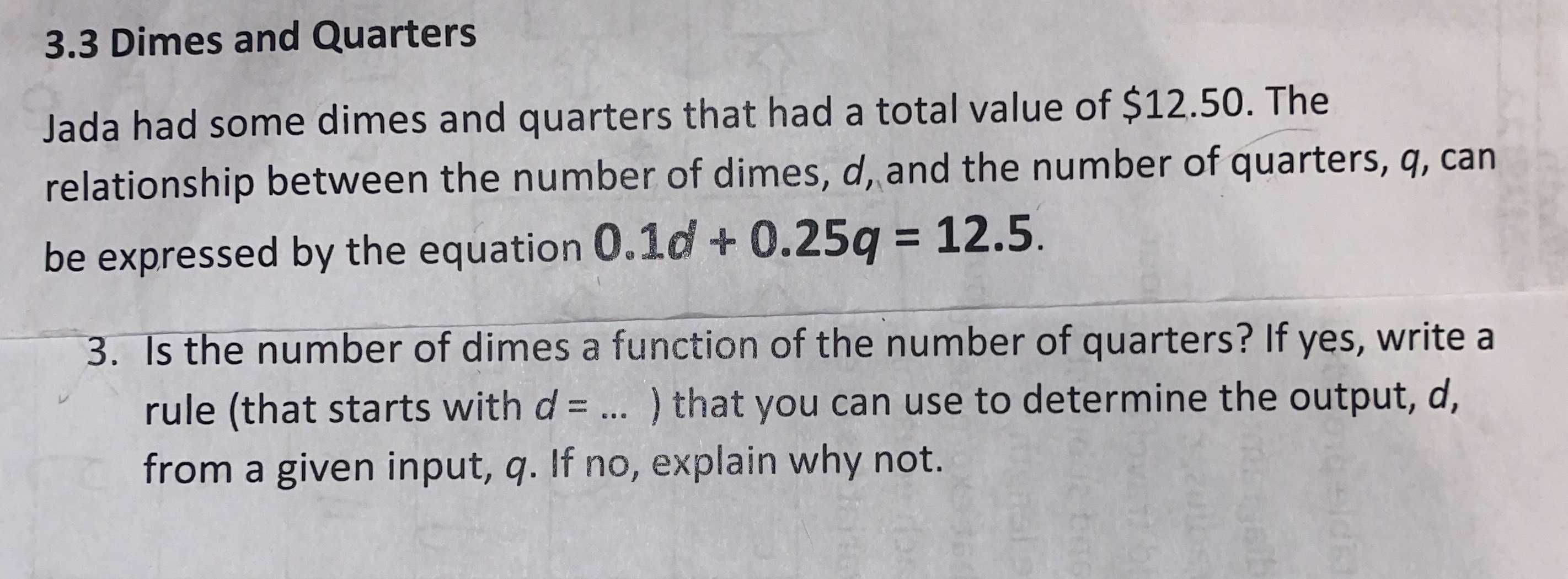### ¿Todavía tienes preguntas de matemáticas?

Pregunte a nuestros tutores expertos
Algebra
Pregunta$$3.3$$ Dimes and Quarters

Jada had some dimes and quarters that had a total value of $$\ 12.50$$ . The relationship between the number of dimes, $$d$$ , and the number of quarters, $$q$$ , can be expressed by the equation $$0.1 d + 0.25 q = 12.5$$ .

3. Is the number of dimes a function of the number of quarters? If yes, write a rule (that starts with $$d = \ldots .$$ ) that you can use to determine the output, $$d$$ , from a given input, $$q$$ . If no, explain why not.